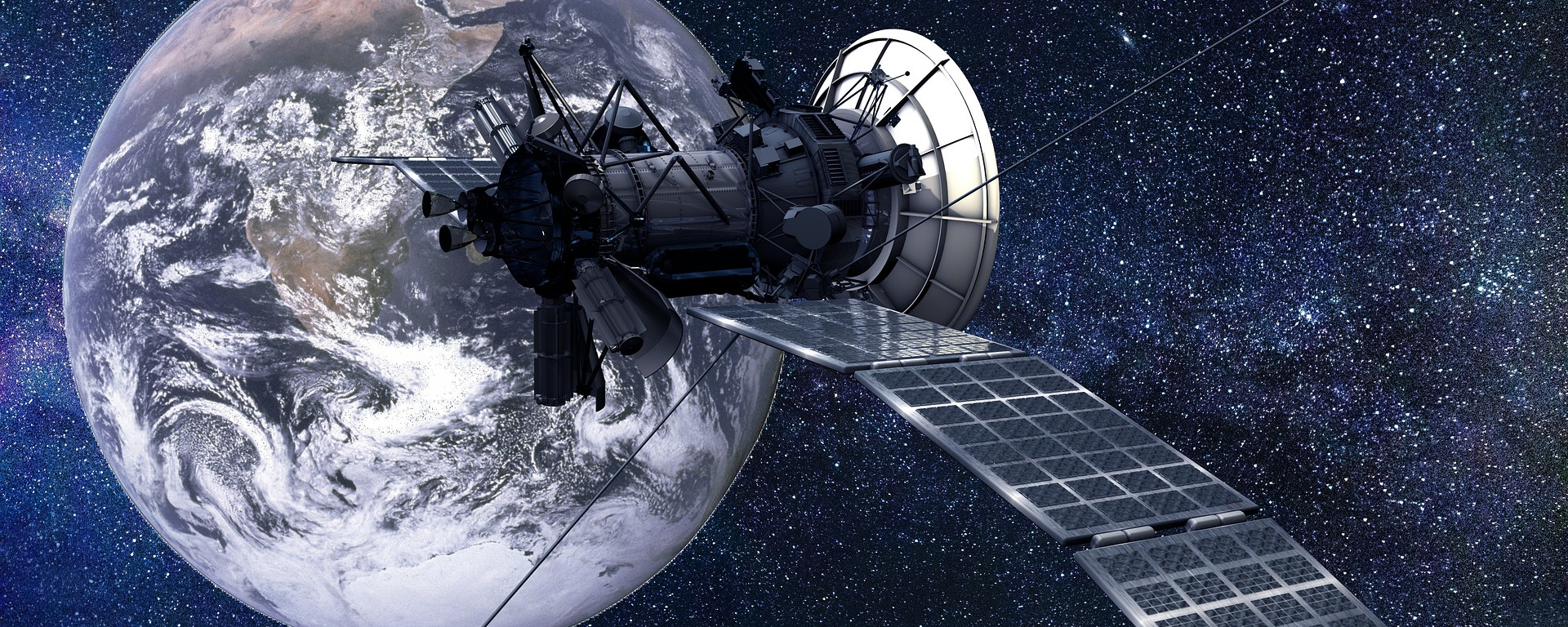# Gravity Assist: the Planetary Slingshot

How does a gravity assist, or slingshot, work exactly for a spacecraft skimming around a planet? Where does the momentum gain come from? Continue reading Gravity Assist: the Planetary Slingshot# Heisenberg’s uncertainty principle

Heisenberg’s uncertainty principle is famous in quantum mechanics. However, it doesn’t have its roots in quantum mechanics. Let’s look at Fourier transform pairs. Continue reading Heisenberg’s uncertainty principle# Simple problems on relativistic energy and momentum

We will focus on a few simple problems where we will manipulate Einstein’s equations for relativistic energy and momentum. Continue reading Simple problems on relativistic energy and momentum# Simple problems on relativistic energy and momentum

We will focus on a couple of simple problems where we will manipulate the equations for relativistic energy en momentum. Continue reading Simple problems on relativistic energy and momentum